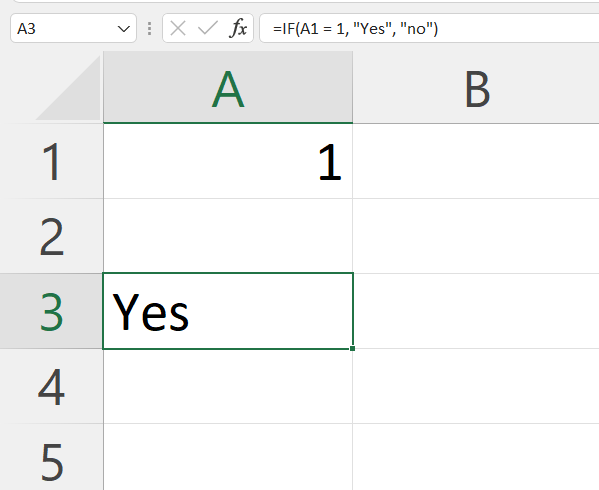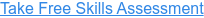Back to Blog# How to use Excel's IF Function

Published
May 21, 2021 10:47:00 AM

Returns one value for a TRUE result, and another for a FALSE result

#### What does Excel's IF function do?

The IF function is one of Excel's logical functions.

The IF function performs a logical test (that is to say - is something true or false) and then returns the value you have provided if the result is TRUE or the value you have provided if the result is FALSE.

The IF function is one of the most popular functions in Excel.

In financial modelling it is often used to model business logic based on 1, 0 flags.

#### Here's a simple example

Let's take a look at a simple example of the IF function.

We would like to check is the value in A1 is the value one. If its is 1 then we would like to return "Yes", if it isn't then we would like to return "No".

#### =IF(A1 = 1, "Yes", "No")#### What does that mean in plain English?

The best way to understand the IF function is to replace the first comma with the word “then” and the second comma with the word “Otherwise”:

If the value in cell A1 is equal to 1, then return “Yes”, otherwise return “No”.

#### =IF(logical test, value if true, value if false)

Logical test – A question resulting in the answer TRUE or FALSE

Value if true – What to return if the answer to the logical test is TRUE

Value if false – What to return if the answer to the logical test is FALSE

#### What to consider when using the IF function in your financial model

• Excel will allow you to nest up to 64 different IF functions. I strongly recommend avoiding nesting IFs wherever possible as formulae with nested IF functions can become incredibly difficult to understand, audit and maintain.
• Instead I recommend that you separate your formula logic onto multiple rows and use the IF function to create 1 or 0 flags.
• XLOOKUP or INDEX MATCH can often provide a clearer and simpler solution to challenges often solved by nested IFs.
• The SWITCH function (2016) provides a simpler alternative to nested IFs.
• The IFS function (2019) was developed to avoid the need for nested IFs.

Read more about the IF function on the Microsoft support page here.

#### Take our free 30-question skills assessment

See where you stand with your Excel, financial modelling, data visualisation & analytics, and modelling tech skills